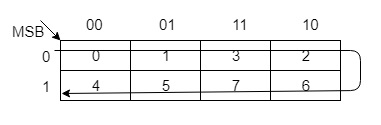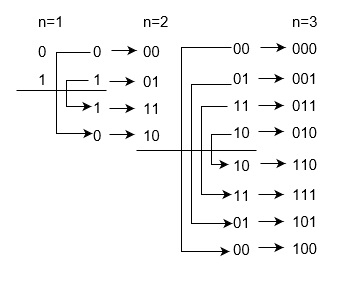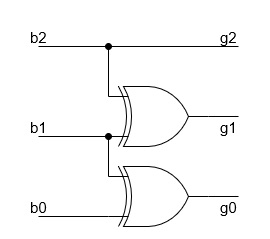# Conversion of Binary to Gray Coden

The reflected binary code or Gray code is an ordering of the binary numeral system such that two successive values differ in only one bit (binary digit). Gray codes are very useful in the normal sequence of binary numbers generated by the hardware that may cause an error or ambiguity during the transition from one number to the next. So, the Gray code can eliminate this problem easily since only one bit changes its value during any transition between two numbers.

## Conversion of Binary to Gray Code

Gray codes are used in rotary and optical encoders, Karnaugh maps, and error detection. The hamming distance of two neighbours Gray codes is always 1 and also first Gray code and last Gray code also has Hamming distance is always 1, so it is also called Cyclic codes.

Using Karnaugh (K) - map −You can construct Gray codes using other methods but they may not be performed in parallel like given above method. For example, 3 bit Gray codes can be contracted using K-map which is given as following below:Decimal
Binary
Gray Code
0
000
000
1
001
001
2
010
011
3
011
010
4
100
110
5
101
111
6
110
101
7
111
100

Using Reflect and Prefix method

n-bit Gray code can be generated recursively using reflect and prefix method which is explained as following below.

• Generate code for n=1: 0 and 1 code.
• Take previous code in sequence: 0 and 1.
• Add reversed codes in the following list: 0, 1, 1 and 0.
• Now add prefix 0 for original previous code and prefix 1 for new generated code: 00, 01, 11, and 10.

Therefore, Gray code 0 and 1 are for Binary number 0 and 1 respectively. Gray codes: 00. 01, 11, and 10 are for Binary numbers: 00, 01, 10, and 11 respectively. Similarly you can construct Gray code for 3 bit binary numbers:Using Exclusive-Or (⊕) operation −

This is very simple method to get Gray code from Binary number. These are following steps for n-bit binary numbers −

• The most significant bit (MSB) of the Gray code is always equal to the MSB of the given Binary code.
• Other bits of the output Gray code can be obtained by XORing binary code bit at the index and previous index.

For example, for 3-bit binary number, let Binary digits are b2 , b1 , b0, where b2 is the most significant bit (MSB) and b0 is the least significant bit (LSB) of Binary. Gray code digits are g2 , g1 , g0, where g2 is the most significant bit (MSB) and g0 is the least significant bit (LSB) of Gray code.

Binary
bbb0
Gray Code
ggg0
000
000
001
001
010
011
011
010
100
110
101
111
110
101
111
100

Therefore, you solve boolean expression using k-map, you will get g2=b2, g1=b1⊕b2, and g0=b0⊕b1.Similarly, you can convert n-bit (bnb(n-1)...b2b1b0) Binary number into Gray code (gng(n-1)...g2g1g0). For least significant bit (LSB) g0=b0⊕b1, g1=b1⊕b2, g2=b1⊕b2 , …. g(n-1)=b(n-1)⊕bn, gn=bn.

Example −Convert Binary number 111010 into Gray code.

So, according above algorithm,

g0=b0⊕b1 = 0⊕1 = 1

g1=b1⊕b2 = 1⊕0 = 1

g2=b2⊕b3 = 0⊕1 = 1

g3=b3⊕b4 = 1⊕1 = 0

g4=b4⊕b5 = 1⊕1 = 0

g5=b5 = 1 = 1

So, Gray will be 100111.